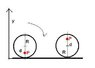# Polar co-ordinates problem.

• MarcL
In summary, the question asks what happens to the particle when the wheel rotates, and it is explained that it remains at a fixed point on the spoke.

#### MarcL

Hum I don't know if it is the right section, I mean I am taking cal 3 but this doesn't really uses calculus... anyway I'll post it here due to the nature of my class.

## Homework Statement

A bicycle wheel has radius R. Let P be a point on the spoke of a wheel at a distance d from the center of the wheel. The wheel begins to roll to the right along the the x-axis. The curve traced out by P is given by the following parametric equations:

x=15(θ)-6sin(θ)
y=15-6cos(θ)

## Homework Equations

When it goes cw θ< 0

## The Attempt at a Solution

I really don't know where to start, the wheel makes a cycloid shape but I don't know how to start solving for it, this question just seems so odd to me, a hint in the right direction would be appreciated?

MarcL said:
Hum I don't know if it is the right section, I mean I am taking cal 3 but this doesn't really uses calculus... anyway I'll post it here due to the nature of my class.

## Homework Statement

A bicycle wheel has radius R. Let P be a point on the spoke of a wheel at a distance d from the center of the wheel. The wheel begins to roll to the right along the the x-axis. The curve traced out by P is given by the following parametric equations:

x=15(θ)-6sin(θ)
y=15-6cos(θ)

## Homework Equations

When it goes cw θ< 0

## The Attempt at a Solution

I really don't know where to start, the wheel makes a cycloid shape but I don't know how to start solving for it, this question just seems so odd to me, a hint in the right direction would be appreciated?

What's the question?

What does "When it goes cw θ< 0" mean?

How does d figure into the problem?

It would be helpful if you provided the complete problem statement, exactly as written.

That is the completely problem statement, there isn't more or less. What is asked is what I wrote up there. I have to find the d and R. How? I got no idea, the bicycle role towards the right axis, so the angle from point P on the wheel (arbitrarily) will go Clockwise and therefore the P(R,θ) will have θ<0 because it is moving clockwise ( property of polar co-ordinate). There isn't much else I can provide you with...

Before I provide any help I would like to double-check my understanding of the set up for the problem.

Suppose there is a bicycle wheel of radius R, There is a particle on a spoke of the wheel at a distance d from the centre (a spoke connects the centre to the edge of the circle entirely in the radial direction). Given the parametric equations provided I assume that the particle remains at a fixed point on this spoke as the wheel rotates - is this correct or is the particle free to move radially along the spoke?. The wheel begins to rotate clockwise (ie. observe the bike moving to the right) and we say that the angle ##\theta## is less than 0 during this motion (ie. ##\theta_{i} = 0## and ##\theta_{t} < 0##. Is this the correct interpretation?

Remember we have one of the fundamental equations relating Cartesian and Polar coordinates:

$$r^{2} = x^{2} + y^{2}$$

Draw a picture of the wheel as it rolls on the ground. What is the lowest and highest y coordinate of P in terms of the radius and d? At what angle is y maximum and at what angle is it minimum?

ehild

#### Attachments

•wheelpoint.JPG
6.4 KB · Views: 449